In the picture, Lines L1 and L2 are parallel, Lines L3 and L4 are parallel, L1 and L3 intersect on the circle, and L2 passes through the center of the circle. The angle theta equals pi/3, and radius R = 6. Find the area of the region A, which lies outside the circle and is bounded within the three line segments. Round your answer to the nearest hundredth. The answer is 29.72. Help!!

Can you upload a picture? I can’t draw this from your description alone. Put it in the comments below this post, and then I’m happy to help.

Update: thanks for uploading a pic. Here’s a version I whipped up that’s a little easier to read—hopefully I got all the important bits right. Note that I just put 60° for the angle instead ofjust to keep things a little simpler.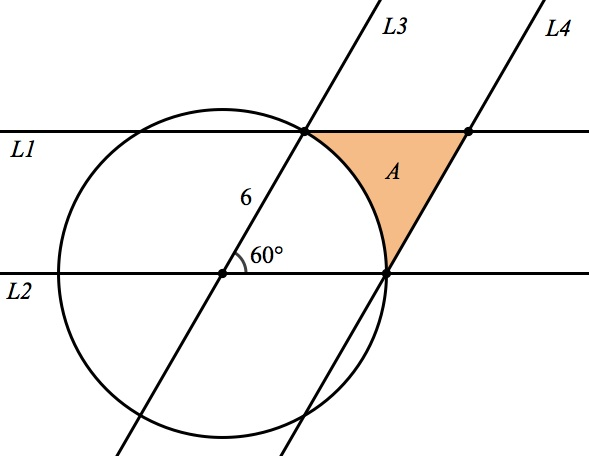Here’s the first thing you want to recognize: the quadrilateral we have here is a parallelogram with an angle of 60°. That means its other three angles will be 60°, 120°, and 120°.

We can calculate the area of a parallelogram by multiplying its base and its height. We know the base is the radius, 6. We need to calculate the height, but that’s easy because if we draw in the height in the right place, we make a 30°-60°-90° triangle! Here’s where I make my usual reminders that the special right triangle ratios should always be at the front of our minds.So the height of the parallelogram is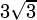. Let’s calculate the area of the parallelogram: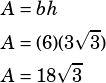Now we just need to subtract the area of the sector (AKA pizza slice) from that.Since the circle’s radius is 6, its area is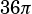. 60° is one sixth of the circle, so the sector we need to pull out is one sixth of the circle’s full area.Now we have what we need to solve! The answer will be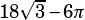. Put that in your calculator to get a decimal approximation and you’ve got your answer–I’m getting 12.33. Is that what you were getting?

The book you’re working from is wrong. Here’s a sanity check: the area of A should be a bit less than half the area of the parallelogram. We already know the whole parallelogram has an area of, so half that will be.Prep Wright says:

Sorry – it’s upside down : /
One of my students found it in an SAT book & I was completely flumoxed! Actually, I thought I knew how to do it (by subtraction as I would with shaded region problems), but I didn’t get the right answer. Thank you!Mike McClenathan says:Prep Wright says: